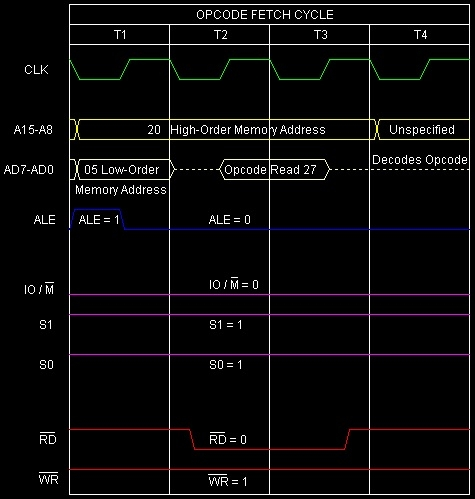# DAA instruction in 8085 Microprocessor

Let us consider we want to add two decimal numbers 38 and 45. They will be represented in BCD as 0011 1000 and 0100 0101. The addition results in 0111 1101. But the answer will be incorrect if we want to interpret this result as a BCD number. The result will be not only incorrect but also illegal as 1101, which we obtained as the last nibble in the answer is not a valid BCD number. Here, in such situations, we can use DAA to have the BCD sum as outcome. All that is required to be done is to add the BCD numbers and store the result in A, and then execute the DAA instruction.

Here is the detailing of the calculations −

  38 ---> 0011 1000
+ 45 ---> 0100 0101
----     ---------
83     0111 1101
---- ----
7    D

The working of DAA instruction depends on the contents of the AL register, Cy, and AC flags. In effect, it adds 00H, 06H, 60H, or 66H to Accumulator so as to get the correct BCD answer in the Accumulator. So here is the illustration of the remedial actions against the previous example −

  38 ---> 0011 1000
+ 45 ---> 0100 0101
----      ---------        1
83      0111 1101           0111 1101
---- ----              + 0110 (06H)
7    D           ---------
1000 0011 ---> 83 (Decimal sum)

As the summary of all related rules are listed below −

• If the LS hex digit in A is <= 9 and AC flag is 0, the LS hex digit value will not be altered.

• If the LS hex digit is >9, or if AC flag is set to 1, it adds 6 to the LS hex digit of A. If carry results, then it increments the MS hex digit if this addition resulted in a carry to the MS digit position. In this process, the Cy flag will be set to 1 if the MS hex digit was incremented from F to 0.

• If the MS hex digit is <= 9 and Cy flag is 0, the MS hex digit will not be altered, and Cy flag is reset to 0.

• If the MS hex digit is > 9, or if Cy flag is set to 1, it adds 6 to the MS hex digit of A and sets Cy flag to 1.

Note that for decimal subtraction DAA instruction cannot be used. Due to unavailability of decimal subtraction in Intel 8085 instruction set, a series of instructions are to be executed to perform decimal subtraction.

Let us consider some examples −

### Example – 1

2000
3E
MVI A, 38H
A ← 38H
2001
38

38H as operand
2002
06
MVI B, 45H
B ← 45H
2003
45

45H as operand
2004
80
A ← A + B; A <- 38H + 45H; A ← 7DH
2005
27
DAA
A ← 83H (Decimal Sum), S = 1,Z = 0,Ac = 1,P = 0,Cy = 0 06H got added with the Accumulator content. As Cy=0, so interpreted result is 83 in decimal

### Example – 2

2000
3E
MVI A, 38H
A ← 38H
2001
38

38H as operand
2002
06
MVI B, 41H
B ← 41H
2003
41

41H as operand
2004
80
A ← A + B; A <- 38H + 41H; A <- 79H
2005
27
DAA
A ← 79H (Decimal Sum), S = 0,Z = 0,Ac = 0,P = 0,Cy = 0 00H got added with the Accumulator content. As Cy = 0, so interpreted result is 79 in decimal

### Example – 3

2000
3E
MVI A, 83H
A ← 83H
2001
83

83H as operand
2002
06
MVI B, 54H
B ← 54H
2003
54

54H as operand
2004
80
A ← A + B; A ← 83H + 54H; A ← D7H
2005
27
DAA
A ← 37H (Decimal Sum), S=0,Z=0,Ac=0,P=0,Cy=1 60H got added with the Accumulator content. As Cy=1, so interpreted result is 137 in decimal

### Example – 4

2000
3E
MVI A, 88H
A ← 88H
2001
88

88H as operand
2002
06
MVI B, 44H
B ← 44H
2003
44

44H as operand
2004
80
A ← A + B; A <- 88H + 44H; A ← CCH
2005
27
DAA
A ← 32H (Decimal Sum), S=0,Z=0,Ac=0,P=0,Cy=1 66H got added with the Accumulator content. As Cy=1, so interpreted result is 132 in decimal

The timing diagram against this instruction DAA execution is as follows −Summary − So this instruction DAA requires 1-Byte, 1-Machine Cycle (Opcode Fetch) and 4 T-States for execution as shown in the timing diagram.